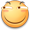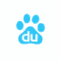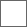• 48回复贴，共1

### 我发表了一篇图片贴，大伙来看看吧~5+（5+4+10）+2=26 不解释,5+2×5＝155+10=15✘3.5=52.5

155+（5+4+10）×2
=5+19×2
=5+38
=435+（4+5+10）*2=43。。。我的是正确的。231250 好吗谢谢 鞋子1个10 人是5 但是那个人穿了鞋子等于10+15×2=50#### 扫二维码下载贴吧客户端